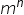Maths-
General
Easy

###Hint:

In this question, the number of onto functions that can be defined from A= {a, b, c, d, e} to {1,2}. Formula to find the number of onto functions from set A to set B We have to find the number of onto functions from a set A with n number of elements to set B with m number of elements. Total number of functions from A to B =.

## Book A Free Demo+91